# Test: Inequalities- 1

## 20 Questions MCQ Test General Test Preparation for CUET | Test: Inequalities- 1

Description
Attempt Test: Inequalities- 1 | 20 questions in 40 minutes | Mock test for Quant preparation | Free important questions MCQ to study General Test Preparation for CUET for Quant Exam | Download free PDF with solutions
QUESTION: 1

### If x+5 < 6, which of the following inequalities best represents the range in which x lies?

Solution:

Given inequality is x+5 < 6

This is a linear inequality in a single variable. So it can be easily solved to obtain the range of x by keeping the variable on one side and taking the constant values to the other side of the inequality.

Now to make sure that there is only “x” on the LHS, let us add “-5” on both sides of the inequality.

(Remember that adding the same quantity on both sides of an inequality doesn’t change its sign.)

• x + 5 – 5 < 6 – 5
• x < 1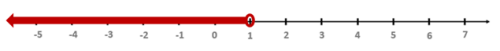QUESTION: 2

### If 21x-42 ≤ 84, then which of the following inequalities is correct?

Solution:

Given inequality is

21x-42 ≤ 84

We know that multiplying both sides of the inequality with a positive number doesn’t change the sign of the inequality.

Multiplying both sides with 1/21, we get

x – 2 ≤ 4

• x – 2 + 2 ≤ 4 + 2
• x ≤ 6

QUESTION: 3

### Which of the following is equivalent to the pair of inequalities x-3 ≤ 5 and x+1 > 6?

Solution:

The first inequality is x – 3 ≤ 5

This is a linear inequality in a single variable. So it can be easily solved to obtain the range of x by keeping the variable on one side and taking the constant values to the other side of the inequality.

Let us add 3 on both sides to make sure that only x remains on the left hand side.

• x – 3 + 3 ≤ 5 + 3
• x ≤ 8The second inequality is x+1 > 6

Let us add -1 on both sides to make sure that only x remains on the left hand side.

• x+1-1 > 6-1
• x > 5Now combining these two:You can observe the following:

1. The overlapping region is between 5 and 8.
2. There is a hole at 5
3. There is a full circle at 8.

Therefore we have 5 < x ≤ 8

QUESTION: 4

If a = -1/2, which of the following is true?

Solution:

If you observe the question along with the option statements, the question is essentially asking us to find the relation between a, a-1, a-2

So we simply need to find the values of each of these. For this, we are given that a = -1/2

We also know that when we are dealing with negative exponents, a-m = 1/am

Therefore a-1 = 1/a = 1/(-1/2) = -2

Similarly, a-2 = 1/a2 = 1/(-1/2)^2 = 1/(1/4) = 4

Therefore we have,

a = -1/2 = -0.5; a-1 = -2; a-2 = 4;

Among these three numbers, -2 is the lowest and 4 is the highest.

Therefore, if we arrange the given numbers in ascending order, we get:

a-1 < a  < a-2

QUESTION: 5

If z is an integer, find the value of z.

(1) -6z -14 > -4z

(2) -3z + 15 < 42

Solution:

Steps 1 & 2: Understand Question and Draw Inferences

We are given that z is an integer, and we have to find the value of z.

Since there is no other information provided in the question, let’s move on to the analysis of statements I and II.

Step 3: Analyze Statement 1

Statement 1 says: -6z -14 > -4z

Let’s try to solve this inequality:

-6z -14 > -4z

By adding 4z + 14 in both sides of the inequality,

-6z -14 + 4z + 14 > -4z + 4z + 14

-2z > 14

Multiplying both sides by -1/2,

z < -7          (Multiplying both sides by negative sign changes the sign of inequality)

So, statement (1) tells us that

z < -7

Thus, possible values of z are {-8, -9, -10, -11 ……. }

Since there are a number of integers less than -7, so we can’t determine the exact value of z.

Hence, statement (1) is not sufficient to answer the question: What is the value of z?

Step 4: Analyze Statement 2

Statement 2 says:  -3z + 15 < 42

Let’s try to solve this inequality:

-3z + 15 < 42

By subtracting 15 from both sides of the inequality,

-3z + 15 -15 < 42 -15

-3z < 27

Multiplying both sides by -1/3,

z > -9          (Multiplying both sides by negative sign changes the sign of inequality)

So, statement (2) tells us that

z > -9

Thus, possible values of z: {-8, -7, -6, -5 …….}

Since there are a number of integers greater than -9, so we can’t determine the exact value of z.

Hence, statement (2) is not sufficient to answer the question: What is the value of z?

Step 5: Analyze Both Statements Together (if needed)

Since statement (1) and (2) alone are not sufficient to answer the question, let’s analyse both these statements together:

Per statement (1):

z = -8, -9, -10, -11 …….

Per statement (2):

z = -8, -7, -6, -5 …….

Combining both the statements, we get

z = -8

Hence, statement (1) and (2) together are sufficient to answer the question: What is the value of z?

QUESTION: 6

If  0 < jk < 1, which of the following can be true?

Solution:

Step 1: Question statement and Inferences

This question asks which of the following options can be true. So, if we can find any values of j and k such that these values satisfy the given inequality then we can say that this option can be true. The option need not to be true for all the values of j and k.

Now, we are given that 0 < jk < 1 . Let’s draw the range of 0 < jk < 1 on number line.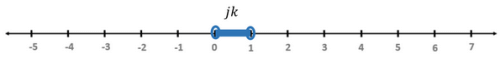This means the product of j and k is a positive number since it is greater than 0.

We know that the product of two numbers is a positive number only if either both the numbers are positive or both the numbers are negative. So, the primary condition that these numbers should fulfill is:

1. Either both j and k are positive
2. Or both j and k are negative

Step 2: Finding required values

Let us look at the answer choices one by one.

1.  j < -1 and k < -1

Here, j < -1 and k < -1 mean that both  &  are negative numbers. Since both  and are negative, these numbers satisfy above criterion.

However, the product of both these numbers will be greater than 1, since their values without sign are greater than 1.

For example:

If j = -1.1 and k = -1.2

The product jk = 1.32

2.  j < -1 and k > 0

This option doesn’t satisfy the primary condition mentioned for j and k. Thus, the product of j and k will not lie in the given range.

3.  j > -1 and k < -1

Here, k < -1 means that  is negative, whereas j > -1  means that  can be negative, positive, or even 0. This may or may not satisfy our criterion. It will depend on what value  assumes.

We need to find such values of j and k that give us 0 < jk < 1

Let’s say j=−1/8

and k = -2

Thus,

jk=(−1/8)∗(−2)=1/4

Hence, the values of j and k per this option give us the value of jk that satisfies the condition stated in the question statement.

4. j > 1 and k < -1

This option doesn’t satisfy the primary condition mentioned for j and k. Thus, the product of j and k will not lie in the given range.

5. j > 1 and k >1

Here,   j > 1 and k >1  mean that both  &  are positive numbers. Since both  and are positive, these numbers satisfy the primary condition.

However, the product of both these numbers will be greater than 1, since the values of both the numbers are greater than 1. The product of two numbers will always be greater than 1, if both the numbers are greater than 1.

For example:

If j = 1.3 and k = 1.4

The product jk = 1.82

Step 3: Calculating the final answer

So, only option C gives us such values of j and k that make the product jk greater than 0 and smaller than 1.

QUESTION: 7

Is m < m2 < m3 , for all real values of m?

(1) m < m3

(2) m3 > m2

Solution:

Steps 1 & 2: Understand Question and Draw Inferences

We are given that m is a real number, and we have to find whether m < m2 < m3 is true or not.

Now, let’s analyze all the possible cases for the values of m:

1.  If, m = 0 or 1,

Then, m = m2 = m3

In this case, all values will be either 0 or 1.

2. If m > 1,

Then, m < m2 < m3

For example, if m =2, then m < m2 < m3= 2 < 4 < 8.

3. If m < -1,

Then, m< m < m2

For example, if m = -2, then m = -2; m2 = 4; m3 = -8

4. If 1 > m > 0,

Then, m< m< m

For example if m=1/2

, then m=-/2,  m2=1/4 , and m3=1/8

5. If 0 > m > - 1,

Then, m < m< m2

For example, if m=−12

, then  m=−1/2 , m2=1/4, and m3=−1/8

Now, we have to find out whether  m < m2 < m?

We have seen that this happens only in case II above. Therefore, what we actually need to find is if m is greater than 1 or not.

Step 3: Analyze Statement 1

Statement 1 says: m < m3

Per the analysis performed in step 1 and 2, we know that m < mis possible in two cases: Case II and case V.

That is, if m > 1 or if 0 > m > - 1

Thus, we can’t determine for sure whether m > 1 or not.

Hence, statement I alone is not sufficient to answer the question: Is m < m2 < m?

Step 4: Analyze Statement 2

Statement 2 says:  m3 > m2

Per the analysis performed in step 1 and 2, we know that m< mis possible only in case II.

Thus, per case II,

m > 1 and

m < m2 < m3

Hence, statement (2) alone is sufficient to answer the question: Is m < m2 < m?

Step 5: Analyze Both Statements Together (if needed)

Since statement (2) alone is sufficient to answer the question, we don’t need to perform this step.

QUESTION: 8

The sum of the ages of Abe, Bianca and Carey is greater than 100 years. 10 years from now, the sum of the ages of Abe and Bianca will be less than 60. Which of the following could be Carey’s current age?

Solution:

Let’s suppose the ages of Abe, Bianca and Carey are A, B and C years respectively.

We are given that sum of the ages of Abe, Bianca and Carey is greater than 100 years.

• A + B + C > 100

Writing this in standard form, we get:

A + B + C – 100 > 0 (Let’s call this I)

It is also given that 10 years from now the sum of ages of Abe and Bianca will be less than 60.

10 years from now, Abe’s age = A + 10 & Bianca’s age = B + 10

Therefore, it is given that: (A + 10) + (B + 10) < 60

• A + B + 20 < 60
• A + B < 40
• A + B – 40 < 0 (Let’s call this II)

Let is subtract II from I to get an inequality exclusively in C.

I: A + B + C – 100 > 0

II: A + B – 40 < 0

-II: -A -B + 40 > 0

Observe that both I and –II have the same inequality sign.

So we can safely add them.

Therefore, we get:

I – II: A + B + C – 100 – A – B + 40 > 0

• C – 60 > 0
• C > 60

Therefore Carey’s current age is greater than 60 years.

Among the given options, there is only one option (70) which is greater than 60.

QUESTION: 9

If x < 2, can y be equal to 2?

(1)  x – y > 3

(2)  x -2y -4 = 0

Solution:

Steps 1 & 2: Understand Question and Draw Inferences

Given that x < 2.

• x – 2 < 0  (Let’s call this I)

We need to find if y can be equal to 2.

Step 3: Analyze Statement 1

Statement 1 says: x – y > 3

This is not in the standard form since the RHS is not zero. So let us write this in the standard form

x – y -3 > 3 – 3

• x – y -3 > 0 (Let’s call this II)

We can eliminate x by subtracting II from I.

I: x – 2 < 0

II: x – y – 3 > 0

-II: -x + y + 3 < 0

Since both I and –II have the same inequality sign, we can add them.

Therefore I – II gives: y + 3 – 2 < 0

• y + 1 < 0
• y < -1

Therefore all real numbers less than -1 are the possible values of y. Hence y can't be equal to 2

Therefore statement 1 is sufficient to arrive at the answer.

Step 4: Analyze Statement 2

Statement 2 says x – 2y – 4 = 0.

Since we need to find the value(s) of y, let us represent x in terms of y.

So let us keep x in LHS and take everything else to the RHS.

• x = 2y + 4

Using this in I, we get:

(2y + 4) – 2 < 0

• 2y + 2 < 0
• y + 1 < 0
• y < -1

Therefore all real numbers less than -1 are the possible values of y. Hence y can't be equal to 2.

Therefore statement 2 is sufficient to arrive at the answer.

Step 5: Analyze Both Statements Together (if needed)

We arrived at unique answers in statement 1 and statement 2. Therefore this step is not needed.

QUESTION: 10

If x and y are negative integers, is x + y ≤ -4?

(1)  (x – 1)(y + 3) = 0

(2)  x < y

Solution:

Steps 1 & 2: Understand Question and Draw Inferences

The question is a Yes/No type of DS question. There are two important pieces of information –

• x and y are both negative
• x and y are both integers.

This means that the maximum value of x and y is -1 each.

The question asks us if the sum of these two negative integers is less than OR equal to -4.

Please notice the possibility of x + y being equal to -4 also.

Step 3: Analyze Statement 1

Either x – 1 = 0 or y + 3 = 0

• In other words, x = 1 or y = -3
• x = 1 is rejected because x must be negative.
• y = -3 is the only solution.

Let’s check if x + y ≤ -4.

• y = -3 and the maximum value of x = -1.
• x can be -1, -2, -3 and so on.

x + y can be -4, -5, -6 and so on.

• Is x + y ≤ -4?
• Yes!

SUFFICIENT.

Step 4: Analyze Statement 2

x is a smaller negative integer than y.

• If y = -1, then x = -2, -3, -4 and so on.
• If we add x and y, we would notice that
• x + y = -3, -4, -5 and so on.

From these values, we see that

• x + y can be less than -4 or can be more than -4.

INSUFFICIENT.

Step 5: Analyze Both Statements Together (if needed)

We get a unique answer in step 3, so this step is not required

QUESTION: 11

Given 2x + 3y + 4z > 2, can z be equal to zero?

(1)  4x + 6y < -3

(2)  9y + 12z > 4

Solution:

Steps 1 & 2: Understand Question and Draw Inferences

Given inequality is 2x + 3y + 4z > 2 (Let’s call this A)

We need to find if z can be equal to zero.

So we need to find a relation between x, y and z suitable enough to draw some inference regarding the range of z or we need to find the range of z.

Step 3: Analyze Statement 1

Statement 1 says:

4x + 6y < -3 (Let’s call this B)

Observe that we can eliminate x and y by doing 2A – B

2A: 4x + 6y + 8z > 4

-B: -4x – 6y > 3

Notice that we can add these two inequalities because both of them have the same inequality sign.

Therefore 2A – B: 4x + 6y + 8z – 4x – 6y > 4 + 3

• 8z > 7
• z > 7/8

Since z is greater than a positive number, z is always greater than zero.

In other words, z can never be equal to zero.

Therefore statement I is sufficient to arrive at a unique answer.

Step 4: Analyze Statement 2

Statement 2 says:

9y + 12z > 4 (Let’s call this C)

Observe that A is a linear inequality in x, y, and z, while C is a linear inequality in y and z.

Therefore it is not possible to get an expression exclusively in z. (Any non-zero linear combination of A and C will always contain x in the expression).

Therefore statement 2 is not sufficient to arrive at a unique answer.

Step 5: Analyze Both Statements Together (if needed)

We already arrived at a unique answer in Step 3. Hence this step is not needed.

QUESTION: 12

There are two classrooms A and B. The sum of the number of students in both classrooms is more than 120. Is the number of students in class B greater than 20?

(1) If number of students in classroom A are doubled and number of students in classroom B are halved, the difference between the number of students in classroom A and B is less than 200.

(2) If 20 students from each classroom leave the school, the sum of number of students in both classes would be more than 80.

Solution:

Steps 1 & 2: Understand Question and Draw Inferences

We are given that the number of students in classes A and B combined is more than 120. We have to find whether the number of students in class B is more than 20.

Let’s say the number of students in class A is x, and the number of students in class B is y.

So, we can say that:

x+y>120

Since there is no other information given in the sentence, let’s move on to the analysis of the statement I.

Step 3: Analyze Statement 1

2x−y/2<200

4x−y<400         ................... (2)

By multiplying inequality (1) with -4, we get:

−4x−4y<−480        .....................(3)

−5y<−80

By multiplying the above inequality with -1, we get:

5y>80y>16

So, y can take any value greater than 16. Thus, we can’t say whether it is greater than 20 or not.

Hence, statement I is not sufficient to answer the question: Is the number of students in class B is more than 20?

Step 4: Analyze Statement 2

Per statement II:

(x−20)+(y−20)>80

x+y>120

This is the same information given in the original sentence. So, from this information we can’t say whether y is greater than 20 or not.

Hence, statement II alone is insufficient to answer the question: Is the number of students in class B is more than 20?

Step 5: Analyze Both Statements Together (if needed)

Since statement I and II alone are not sufficient to answer the question, let’s analyse them together.

However, since statement II provides the same information given in the original sentence, analysing both the statements together is equivalent to analysing statement I alone.

Thus, even both statements combined are not sufficient to answer the question: Is the number of students in class B is more than 20?

The correct answer is: Statements (1) and (2) TOGETHER are NOT sufficient.

QUESTION: 13

If x and y are non-negative integers, x+y<11,andx−y>8, which of the following must be true for all the qualified values of x?

Solution:

Step 1: Question statement and Inferences:

We are given two linear inequalities:

x + y < 11 ............................(1)

x -  y > 8 .............................(2)

where x and y are non-negative integers.

We have to find the option that is true for all the qualified values of x. Now, if we look at the options we get to know that we have to find the range of y. So, we need to eliminate the variable x from the inequalities so that we can get an inequality in x only.

To perform addition or subtraction options on the inequalities above, first we need to convert them into inequalities with the same sign.

So, by multiplying (2) with -1, we get:

- x + y < - 8 .......................(3)

Also, given that x and y are non-negative integers.

This implies, x and y can be 0 or positive integers.

Step 2: Finding required values

y + y < 3

y<32

We know that y is a non-negative integer. So, the possible values of y are only 0 and 1.

Here, it seems that the answer should be option D. However, there is one more condition mentioned in the question. We have to find the value of y for all the qualified values of x.

Now, there are two cases:

Case I: y = 0

x + 0 < 11 .............................(1)

x < 11

x - 0 > 8 ...............................(2)

x > 8

So, x can take only two values i.e. 9 and 10.

Case II: y = 1

x + 1 < 11 ..............................(1)

x < 10

x - 1 > 8 ................................(2)

x > 9

So, per this case the value of x lies in between the integers 10 and 9. However, per the question statement x itself is an integer.

So, there is no integer value of x corresponding to y = 1.

Step 3: Calculating the final answer

Hence, the only possible value of y is 0. This condition is satisfied by option E.

QUESTION: 14

If x and y are positive integers such that x/y < (11/y) – 2, and y = 300% of x, which of the following could be a value of y?

Solution:

Given that x and y are positive integers and that x/y < (11/y) – 2 (Let’s call this I)

We are also given that y = 300% of x

• y = 3x (Let’s call this II)

We need to solve this inequality and equation to find a possible value for y.

Step 1: Convert the inequality I into standard form.

Since y is positive, we can multiply it on both sides of the inequality without changing the sign of the inequality.

• x < 11 – 2y

To convert this into the standard form, we need to make the RHS zero.

• x – 11 + 2y < 11 – 2y – 11 + 2y
• x + 2y – 11 < 0

Step 2: Eliminate one variable.

Since we need the value of y, let us express x in terms of y from II

y = 3x

• x = y/3

Using this in the standard form of I, we get:

y/3 + 2y – 11 < 0

Multiplying by 3 on both sides,

y + 6y – 33 < 0

• 7y – 33 < 0
• 7y < 33
• y < 33/7
• y < 4.71 (approx.) (Let’s call this III)

Since y needs to be an integer, y can be 1,2,3,4. However, since y = 3x and x is an integer, the only value of y that satisfies both the conditions (x integer, y integer) is y = 3 and x = 1.

QUESTION: 15

p and q are positive integers such that 2p -10 > -q, and 3q -20 < -p. If m is the minimum possible value of p and n is the maximum possible value of q, then which of the following pairs accurately represents (m, n).

Solution:

Given inequalities are:

2p – 10 > -q and 3q – 20 < -p

The question statement asks us to find the minimum possible value of p and the maximum possible value of q.

So to know more about the range of values p and q can take, let us now try to solve these inequalities.

Step 1: Write the given inequalities in the standard form

2p + q -10 > 0 (Let’s call this I)

p + 3q -20 < 0 (Let’s call this II)

Step 2: Eliminate one variable

We can eliminate p from the inequalities by subtracting 2*II from I.

I: 2p + q -10 > 0

2*II: 2p + 6q – 40 < 0

-2*II: -2p – 6q + 40 > 0

Since I and -2*II have the same inequality sign, we can safely add them.

I – 2*II: 2p + q -10 -2p – 6q + 40 > 0

• -5q + 30 > 0
• -5q > -30
• 5q < 30
• q < 6 (Let’s call this III).

Writing III in standard form: q – 6 < 0.

Step 3: Find the value(s) of the eliminated variable

We can use I and III to find the value of p.

I: 2p + q -10 > 0

III: q – 6 < 0

-III: -q + 6 > 0

Since I and –III have the same inequality sign, we can safely add them.

I – III: 2p + q -10 – q + 6 > 0

• 2p – 4 > 0
• p > 2 (Let’s call this IV)

So from III and IV we know that, p > 2 and q < 6

We are also given that p and q are positive integers.

Combining these two pieces of information, we can infer the following:

1. Since p > 2 and p is a positive integer, the minimum possible value (just greater than 2) p can take is 3.
2. Since q < 6 and q is a positive integer, the maximum possible value (just lesser than 6) q can take is 5

Therefore, m = 3 and n = 5

QUESTION: 16

If (2-x)2 < 9, which of the following expressions is correct?

Solution:

Step 1: Question statement and Inferences

We will solve this quadratic inequality to determine the possible values of x

(2−x)2<9

Step 2 & 3: Simplifying the expression and calculating the final answer

Subtracting 9 from both sides will not change the sign of inequality:

(2-x)2 – 9 < 0

(2-x)2 – 32 < 0

By applying the formula: a2 – b2 = (a-b)(a+b) to the above inequality, we get:

(2-x -3)(2-x+3) < 0

(-x-1)(5-x) < 0

-1(x+1)(5-x) < 0

(x+1)(x-5) < 0

By using the Wavy Line method, we see that the inequality will hold for the part of the wavy line that is below the number line.

So,

-1 < x < 5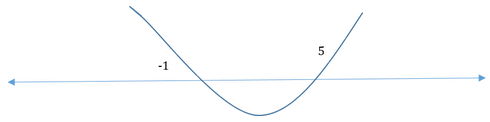QUESTION: 17

If x is a positive integer, what is the value of x?

(1)  (6-2x)(6-x)<0

(2)  (x-5)(x+7)<0

Solution:

Steps 1 & 2: Understand Question and Draw Inferences

Given that x > 0 and that x is an integer.

So any in any interval we consider, we need to consider only the positive integral values of x.

Step 3: Analyze Statement 1

Given (6-2x)(6-x)<0.

• 2(3-x)(6-x)<0
• (3-x)(6-x)<0

The zero points of this inequality are 3 and 6.

If we plot them on a number line and apply the wavy line method: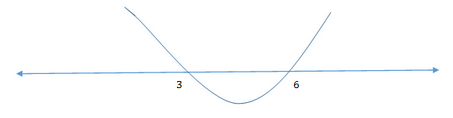We get that the above inequality is satisfied only when 3 < x < 6.

Since x is an integer, the possible values of x are 4 and 5.

Therefore Statement 1 is not sufficient to arrive at a unique answer.

Step 4: Analyze Statement 2

Given (x-5)(x+7)<0.

This is a relatively straightforward inequality and its zero points are -7 and 5.

If we plot them on a number line and apply the wavy line method: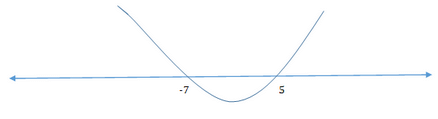We can see that the above inequality is satisfied only when -7 < x < 5.

Since x is a positive integer, the possible values of x are 1, 2, 3, and 4.

Therefore Statement 2 is not sufficient to arrive at a unique answer.

Step 5: Analyze Both Statements Together (if needed)

Now let’s look at what both statements together say.

• Statement 1 says that the possible values of x are 4 and 5.
• Statement 2 says that the possible values of x are 1, 2, 3, and 4.
• Putting statement 1 and statement 2 together, we get x = 4.

Statement 1 and statement 2 together are sufficient to arrive at a unique answer.

QUESTION: 18

If x is a number such that x2−4x+3<0and 2x2−9x+10<0,

which of the following can be the value of x?

Solution:

Step 1: Question statement and Inferences:

We are given two quadratic inequalities:

x2−4x+3<0     ………………. (1)

2x2−9x+10<0  ….…………… (2)

We have to find the value of x that satisfies both the inequalities.

Now, to find the value of the above inequalities, first we need to convert them into standard form with the following two features:

(i)  The coefficient of x2 is positive

(ii) The Right hand side of the inequality is zero

Then, using the wave line method we need to find out values of x that are common to both inequalities.

Step 2: Finding required values

Consider inequality I:

x2−4x+3<0

Since this inequality already follows the standard form, we will factorize it:

(x−1)(x−3)<0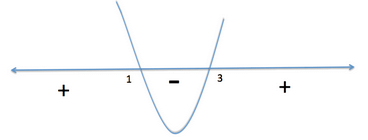Hence,

1<x<3 …………………. (3)

Consider inequality II:

2x2−9x+10<0

Since this inequality already follows the standard form, we will factorize it:

(2x−5)(x−2)<0

(x−5/2)(x−2)<0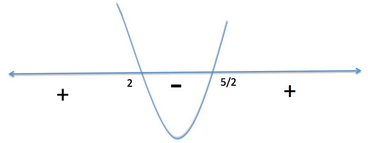Hence,

2<x<5/2 …………………. (4)

Step 3: Calculating the final answer

Now, if we look at the inequalities (3) and (4), we see that the value of x that satisfies both of them will be:

2<x<5/2

That is, 2 < x < 2.5

Out of the given five options, only option A (2.3) falls in this range.

Therefore, the correct answer is: Option (A)

QUESTION: 19

If x is an integer, what is the value of x?

(1)  (2x + 1)(x – 2) < 0

(2)  x2 < 2

Solution:

Steps 1 & 2: Understand Question and Draw Inferences

We need to evaluate the given statements to determine a unique integral value of x.

Step 3: Analyze Statement 1

The inequality (2x+1)(x-2) < 0 is already in factored form.

The zero points are -1/2 and 2

We plot the zero points on the number line and draw a wavy line that passes through the zero points.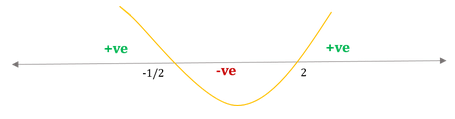The given inequality is negative for

-1/2 < x < 2

The possible integral values of x are 0 and 1. So, not possible to determine a unique solution.

INSUFFICIENT.

Step 4: Analyze Statement 2

x2 < 2

i) Writing the inequality in standard form by subtracting 2 from both sides:

x2 – 2 < 0

ii) Factorizing the inequality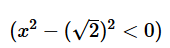=>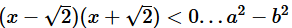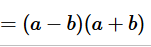iii) Plotting the zero points on the number line

The zero points are -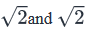iv) Drawing the wavy line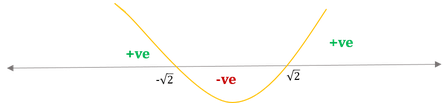The given inequality is negative for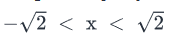Or, approximately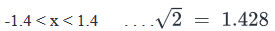The possible integral values of x are -1, 0 and 1.

INSUFFICIENT.

Step 5: Analyze Both Statements Together (if needed)

From Statement 1, the possible integral values of x are 0 and 1

From Statement 2, the possible integral values of x are -1, 0 and

From the two statements together, the possible integral values of x are 0 and 1

We do not have a unique value for x.

INSUFFICIENT.

QUESTION: 20

If y is an integer such that y < -3, which of the following expressions must be correct?

I.  y – y2 + 12 < 0

II.  y2 – y – 12 > 0

III.  2y2 – 3y – 27 > 0

Solution:

Given that y is an integer and y < -3

Therefore the possible values of y are -4, -5, -6, -7 and so on.

Now let’s look at statement I.

Given y – y2 + 12 < 0

Now let us convert it into the standard form by multiplying with -1 on both sides.

• y2 – y – 12 > 0

We can easily see that we can factorize the above expression as follows

• (y – 4)(y + 3) > 0

The zero points of this inequality are -3 and 4.

Plotting these points on the number line and applying the wavy line method: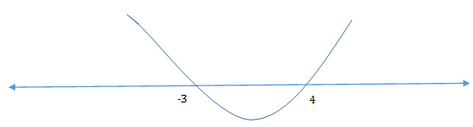We can see that the above inequality holds true (strictly greater than zero) only when y < -3 OR y > 4.

This means that whenever y < -3, Statement I is always true.

Therefore statement I must be true.

Now let’s look at statement II.

Given y2 – y – 12 > 0

This is essentially equivalent to statement I (as seen above).

Therefore statement II must be true.

Now let’s look at statement III.

2y2 – 3y – 27 > 0

The above expression can be easily factorized into (y + 3)(2y – 9)

• (y + 3)(2y – 9) > 0

The zero points of this inequality are -3 and 9/2.

Applying the wavy line method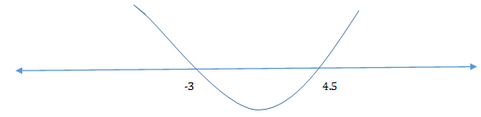We see that the above inequality holds true for y < -3 and for y > 4.5

This means that whenever y < -3, the above inequality holds true.

Therefore statement III must be true.

Therefore all three statements must be true.Use Code STAYHOME200 and get INR 200 additional OFF Use Coupon Code